# Basic Arithmetic : Fractions

## Example Questions

← Previous 1 3 4 5 6 7 8 9

### Example Question #1 : Operations With Fractions

Multiply: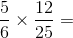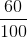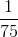Explanation:

Multiply the numerators together, then multiply the denominators together.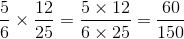To simplfy we can pull out afrom the numerator and denominator, thus canceling them. The following answer is the result: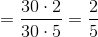### Example Question #1 : Operations With Fractions

In a classroom of 36,of the students are girls. Ifof the girls enjoy eating strawberries, andof the boys also enjoy eating strawberries, how many total students in the class enjoy eating strawberries?Explanation:

First, find out how many girls and boys are in the class.

Multiply 36 byto find the number of girls.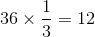Then, subtract the number of girls from the total number of students in the class to find the number of boys.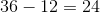Now, we can figure out how many girls enjoy eating strawberries. Multiply the total number of girls by the fraction of girls who enjoy eating strawberries.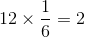Now, do the same with the boys.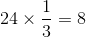Add these two numbers together to get the total number of students who enjoy eating strawberries.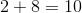### Example Question #1 : Fractions

Solve and simplify.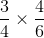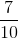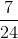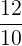Explanation:

When multiplying fractions, you simply multiply the numerators and then multiply the denominators. Then simplify the fraction.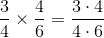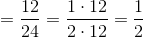(Note: by dividing both our numerator and our denominator by, gives us our final answer of.

### Example Question #2 : Operations With Fractions

Evaluate the following: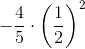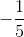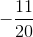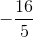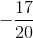Explanation:

This problem involves order of operations.

The correct order is:  Parenthesis, Exponents, Multiplication, Division, Addition, and Subtraction.

Rewrite the exponent and cancel out the 4 on the numerator and denominator.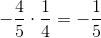### Example Question #1 : Operations With Fractions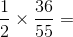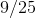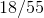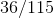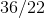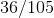Explanation:

To do this problem, simply multiply through (i.e. numerator by numerator and denominator by denominator):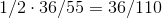And then reduce to find your answer--bothand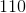are divisible by two.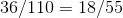### Example Question #1 : Operations With Fractions

Multiply these fractions: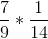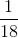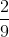Explanation:

When multiplying fractions, all we have to do is multiply the numerators together and multiply the denominators together: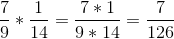Simply the fraction to get the final answer:### Example Question #1 : Operations With Fractions

Multiply these fractions: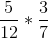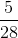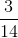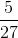Explanation:

When multiplying fractions, all we have to do is multiply the numerators together and multiply the denominators together: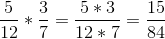Simply the fraction to get the final answer: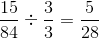### Example Question #1 : Operations With Fractions

Multiply these fractions: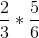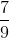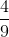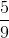Explanation:

When multiplying fractions, all we have to do is multiply the numerators together and multiply the denominators together: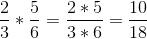Simply the fraction to get the final answer: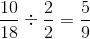### Example Question #1 : Operations With Fractions

Multiply these fractions: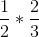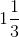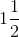Explanation:

When multiplying fractions, all we have to do is multiply the numerators together and multiply the denominators together: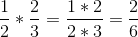Simply the fraction to get the final answer: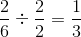### Example Question #10 : Operations With Fractions

Multiply these fractions: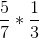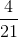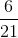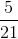Explanation:

When multiplying fractions, all we have to do is multiply the numerators together and multiply the denominators together: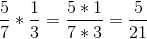← Previous 1 3 4 5 6 7 8 9

### All Basic Arithmetic Resources